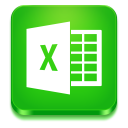24
Views
11
Comments
###### [Excel Package] How to read a formulae from an existing excel file?
QuestionForge component by Carlos Alfaro

Is it possible to read the formula inside a cell instead of the value?

Ex:

In this example, I would read the formula inside B2 (=A2*3) and not the value (6).With the Cell_ReadByIndex Action it is possible to read the value in the cell but not the formula.Manish Gupta

Hello @Pier Paolo Annis

You can create such excel with the below steps -1. Go to the 'File' tab. ...
2. Click on 'Options'.
3. In the left pane, select Advanced.
4. On the right, scroll down to the 'Display options for this worksheet' section.
5. From the drop down, select the worksheet in which you want to show the formulas instead of values.

Then use the excel in OutSystems to get the formulas.Pier Paolo Annis

I tried your solution, but I still cannot read (get) the formula inside the cell.Manish Gupta

What data it is fetching instead of the formula? Please Note the Input Variable DataType should be TextPier Paolo Annis

instead of the formula (=A2*3) is fetching the value calculated (6).

The data type is text.Manish Gupta

Just for a trial, can you bootstrap that Excel sheet once in the OutSystems Entity and see what data it brings there!!

If it brings the calculated value then we will need to look at different approach but if it fetches the formula then we are going in right direction and efforts will be to fetch data as it is.

Please let me know the results. I will also try it at my end once reaching to the desk. (Outside at the moment :p)Pier Paolo Annis

With bootstrap (Import New Entities from Excel..), it created the entity and the structure with the values (Type Number) but not the Formula.

I tried to change the data type to Text (for both entity and structure) before publishing but the result is the same.Manish Gupta

Umm, Okay. I saw you shared the OML and Excel... Have you removed that?

Let me try this in my personal environment once.Pier Paolo Annis

I don't know if you are able to open it.

ExampleExcel.omlManish Gupta

I have done this practically and achieve to get the formula -The Problem Is:

• When we set the Excel to display Formula instead of Value - it shows the formula but that's a view only. In the actual results it is the calculated value. So, the data sent to the entity is Value but No formula.
• It is sending Value instead of formula because of =
• Have you noticed in the formula data it has = as prefix that initiates the Math in excel.
• What I did is
• I replaced the = with "blank" in the excel sheet
• This way I get all the formula in TEXT Field
• Then Importing is OutSystems working fine.Let me know if this has solved your problem.

RegardsPier Paolo Annis

Is there another solution more simply without check every single cell?

Cause in this way, I cannot replace all if I have the formula for example =IF(A1=B1,0,1)Manish Gupta

Yes, you can use Text to Column with the Fix width separation -1. Select Formula Row
2. Go To Data Tab
3. Select Text To Column
4. Opt Fixed Width
5. Click Next1. Click Just After =
2. It will create a Line from where data will be separated
3. Click Next1. Select field
2. Select Text as Format
3. Click Finish

It will separate the first = from Formula and you can use the new row as Formula.Community GuidelinesBe kind and respectful, give credit to the original source of content, and search for duplicates before posting.